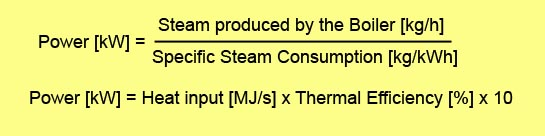# Locomotive Power

## Locomotive Power

Power is defined as “the rate of doing work”. Common units of power in the metric system are Watts (W), kilowatts (kW), Megawatts (MW) and Gigawatts (GW), where 1 Watt = 1 Joule per second = 1 Newton-metre per second. Alternative units of measurement are calories per second and kilo-calories per hour (1 kW = 860 kcal/hr) Common imperial units of power are: Btu per second (1 Btu/s = 1.06 kW) and the Horsepower where 1 (British) hp = 0.746 kW.

Power can also be defined as the multiple of force and speed, from which it can be deduced that a locomotive’s power and tractive effort (TE) are intrinsically related: Power = TE x speed.

Livio Dante Porta liked to define a locomotive’s power in the following thermodynamic terms, as can be found in his papers on “Fundamental Principles of Steam Locomotive Modernization and Their Application to Museum and Tourist Railways” and “Fundamentals of the Porta Compound System for Steam Locomotives“:In reference to the first equation, Porta writes:

“Thus the power is limited by [the amount of steam supplied by] the boiler, while the function of the cylinders is to extract the maximum work from the steam supplied”,

“The second equation shows that the [power] limit is determined by the ability of the boiler to burn as much fuel per hour as possible, but the resulting power is determined by the thermal efficiency.”

A common point of confusion in locomotive terminology is the difference between indicated power and drawbar power, the basic difference being that “indicated power” is the power developed in the cylinders, whereas “drawbar power” is the power delivered at the drawbar.   These terms are defined on separate pages.